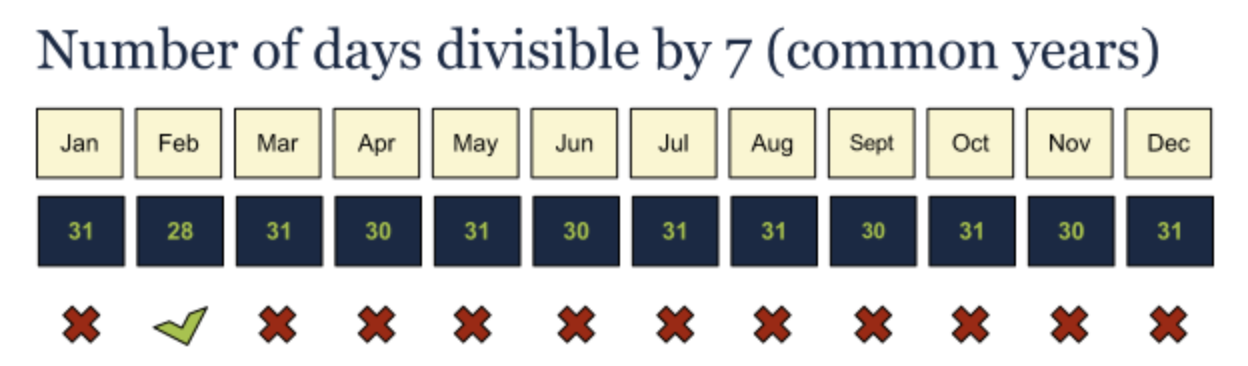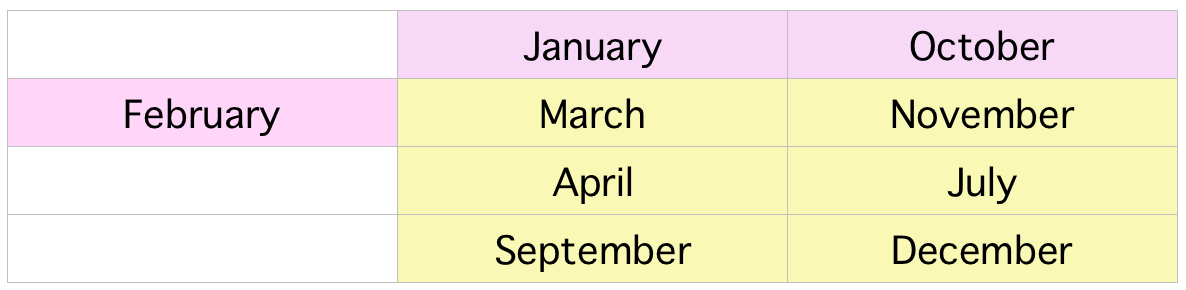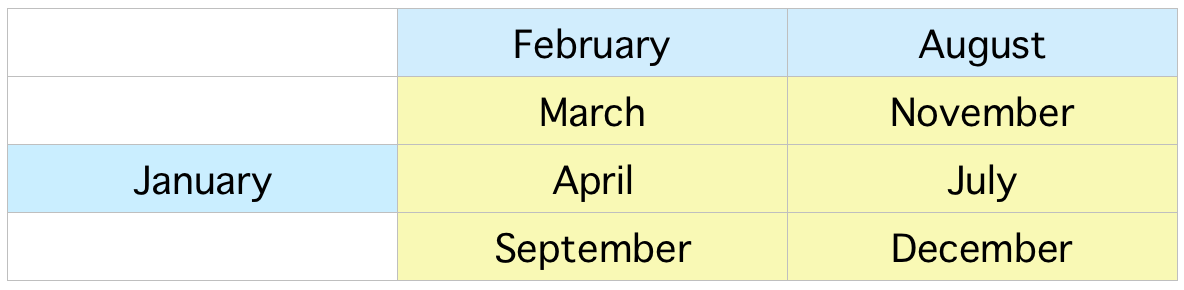# How to determine the day of the week 📅¶

### @vivmarquez¶

#### MSDS 610 - Biz Comm¶

October 1st, 2018

# General idea 🛠️¶

Sunday Monday Tuesday Wednesday Thursday Friday Saturday
0 1 2 3 4 5 6

• Modular arithmetic.

# What do we know about the calendar? 🤔¶

• A common year has 365 days.

• A leap year has 366 days.

• 365 mod 7 = 1.# What else do we know about the calendar? 🤔¶

• In a common year, February has 28 days.

• 28 mod 7 = 0.

• Thus, in a common year, February and March start on the same day.

• Bad news 😫 - Not as easy for the other months!• Good news 😄 - Some months do start the same day as others.

• For example:

- September has 30 days.
- October has 31 days.
- November has 30 days.

- 30 + 31 + 30 = 91.
- 91 mod 7 = 0.

• Therefore, September and December start on the same day! (Always!)

• Corresponding months in a common year:• Corresponding months in a leap year:# The algorithm 🤓¶

In :
# Target date
year = 2018
month = 10
day = 1


### Step #1¶

Get the number for the corresponding century from table:

In :
def get_century(year):
if (1700 <= year and year <= 1799):
return 5

if (1800 <= year and year <= 1899):
return 3

if (1900 <= year and year <= 1999):
return 1

if (2000 <= year and year <= 2099):
return 0

if (2100 <= year and year <= 2199):
return -2

if (2100 <= year and year <= 2199):
return -4

In :
x1 = get_century(year)
x1

Out:
0

### Step #2¶

Get the last two digits from the year:

In :
def get_two_digits_year(year):
return year%100

In :
x2 = get_two_digits_year(year)
x2

Out:
18

### Step #3¶

Divide the number from step two by 4 and get it without the remainder:

In :
def get_no_remainder(x2):
return int(x2/4)

In :
x3 = get_no_remainder(x2)
x3

Out:
4

### Step #4¶

Get the month coefficient:

In :
def get_month(month):
if month == 1 or month == 10:
return 6

if month == 2 or month == 3 or month == 11:
return 2

if month == 4 or month == 7:
return 5

if month == 5:
return 0

if month == 6:
return 3

if month == 8:
return 1

if month == 9 or month == 12:
return 4

In :
x4 = get_month(month)
x4

Out:
6

### Step #5¶

If the year is leap and the month is January or February, return -1. Else, return 0.

In :
def is_leap_year(year):
return year % 4 == 0 and (year % 100 != 0 or year % 400 == 0)

def leap_coefficient(year,month):
if is_leap_year(year) and (month==1 or month==2):
return -1
else:
return 0

In :
x5 = leap_coefficient(year,month)
x5

Out:
0

### Step #6¶

Get the day:

In :
x6 = day
x6

Out:
1

### Step #7¶

Sum the numbers from steps 1 to 6, divide by 7, and take the remainder:

In :
def return_remainder(year,month,day):
x1 = get_century(year)
x2 = get_two_digits_year(year)
x3 = get_no_remainder(x2)
x4 = get_month(month)
x5 = leap_coefficient(year,month)
x6 = day
total = x1 + x2 + x3 + x4 + x5 + x6

In :
return_remainder(year,month,day)

Out:
1

### Step #7¶

Actually find out the day of the week!

In :
def get_day_of_week(year,month,day):
remainder = return_remainder(year,month,day)
days = ["Sunday", "Monday", "Tuesday", "Wednesday", "Thursday", "Friday", "Saturday"]
return f"{year}-{month}-{day} is a {days[remainder]} 🎉"

In :
get_day_of_week(year,month,day)

Out:
'2018-10-1 is a Monday 🎉'In :
from datetime import date
import calendar
my_date = date(2018, 10, 1)
calendar.day_name[my_date.weekday()]

Out:
'Monday'

But how are you going to impress your friends and family? 🤫🤫🤫

# Recap ✍️¶

• Step 1: Get century coefficient. (Tip: 1900's = 1, 2000's = 0) $\Rightarrow$ 0

• Step 2: Get last two digits of the year. $\Rightarrow$ 18
• Step 3: Divide step two by 4. $\Rightarrow$ 4

• Step 4: Get corresponding month coefficient. $\Rightarrow$ 6

• Step 5: If leap and (JAN or FEB) = -1. $\Rightarrow$ 0

• Step 6: Get day. $\Rightarrow$ 1

• Step 7: Total mod 7. $\Rightarrow$ 0+18+4+6+0+1 = 29 mod 7 = 1

# One more 👀¶In :
year = 2022
month = 2
day = 22

In :
get_day_of_week(year,month,day)

Out:
'2022-2-22 is a Tuesday 🎉'

# One more 👀¶In :
year = 2022
month = 2
day = 22

In :
get_day_of_week(year,month,day)

Out:
'2022-2-22 is a Tuesday 🎉'

# Thank you for your attention 🤗¶github.com/vivianamarquez/Determine-Day-of-the-Week